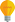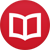# Integral of $\sin^3(x)$

by Jakub MarianTip: See my list of theMost Common Mistakes in English. It will teach you how to avoid mis­takes with com­mas, pre­pos­i­tions, ir­reg­u­lar verbs, and much more.

Integrating the third power of $\sin(x)$ (or any odd power, for that matter), is an easy task (unlike $∫ \sin^2(x)\,dx$, which requires a little trick). All you have to do is write the expression as $\sin(x)⋅(\text{even power of }\sin)$, rewrite the even power using the formula $\sin^2(x) = 1-\cos^2(x)$, and apply the substitution $u = \cos(x)$ (i.e. $du = -\sin(x)dx$). Let’s see it in practice:

$$∫ \sin^3(x)\,dx = ∫ \sin(x)\sin^2(x)\,dx = ∫ \sin(x)(1-\cos^2(x))\,dx = \l.∫ -(1-u^2)\,du\r|_{u = \cos(x)} = -u + \frac{u^3}3 + c = \frac13 \cos^3(x)-\cos(x) + c\,.$$

The same approach works for any odd power of $\sin(x)$ (or $\cos(x)$), only the resulting expressions get slightly more complicated. For example,

$$∫ \sin^5(x)\,dx = ∫ \sin(x)(\sin^2(x))^2\,dx = ∫ \sin(x)(1-\cos^2(x))^2\,dx \\ = ∫ -(1-u^2)^2\,du = ∫ (-u^4+2u^2-1)\,du = -\frac{u^5}5 + \frac{2u^3}3 - u + c \\ = -\frac15 \cos^5(x) + \frac23 \cos^3(x)-\cos(x) + c\,.$$

By the way, I have written several educational ebooks. If you get a copy, you can learn new things and support this website at the same time—why don’t you check them out?